# we have to take the measure of inner scale or outer scale?????????

### Measuring Angles

A protractor is used to measure angles.  In this section, we will consider the use of a protractor that has the shape of a semi-circle and two scales marked from 0º to 180º.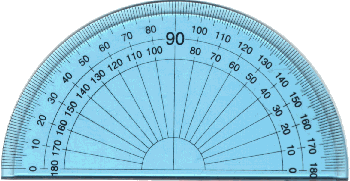The two scales make it easy for us to measure angles facing different ways.To measure the size of angle ABC, place the protractor over the angle so that the centre of the protractor is directly over the angle's vertex, B; and the base line of the protractor is along the arm, BA, of the angle.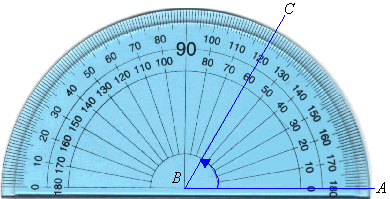We use the inner scale to measure the angle ABC, as the arm AB passes through the zero of the inner scale.  Following the inner scale around the protractor, we find that the other arm, BC, passes through the inner scale at 60º.  So, the size of angle ABC is 60 degrees. We write this as follows: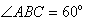To measure the size of angle PQR, place the protractor over the angle so that the centre of the protractor is directly over the angle's vertex, Q; and the base line of the protractor is along the arm, PQ, of the angle.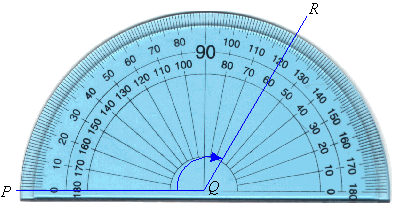We use the outer scale to measure the angle PQR, as the arm PQ passes through the zero of the outer scale.  Following the outer scale around the protractor, we find that the other arm, QR, passes through the outer scale at 120º.  So, the size of angle PQR is 120 degrees.  We write this as follows: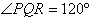• 0

if the angle faces the left side then it is the outer scale.

if the angles faces the right side then it is the inner scale.

• 0

if the angle faces the left side then it is the outer scale.

if the angles faces the right side then it is the inner scale.

• 0

if the angle faces the left side then it is the outer scale.

if the angles faces the right side then it is the inner scale.

• 0

if the angle faces the left side then it is the outer scale.

if the angles faces the right side then it is the inner scale.

• 0

if the angle faces the left side then it is the outer scale.

if the angles faces the right side then it is the inner scale.

• 1

if the angle faces the left side then it is the outer scale.

if the angles faces the right side then it is the inner scale.

• 0

if the angle faces the left side then it is the outer scale.

if the angles faces the right side then it is the inner scale.

• 0

if the angle faces the left side then it is the outer scale.

if the angles faces the right side then it is the inner scale.

• 0

if the angle faces the left side then it is the outer scale.

if the angles faces the right side then it is the inner scale.

• 0

if the angle faces the left side then it is the outer scale.

if the angles faces the right side then it is the inner scale.

• 0

if the angle faces the left side then it is the outer scale.

if the angles faces the right side then it is the inner scale.

• 0
What are you looking for?